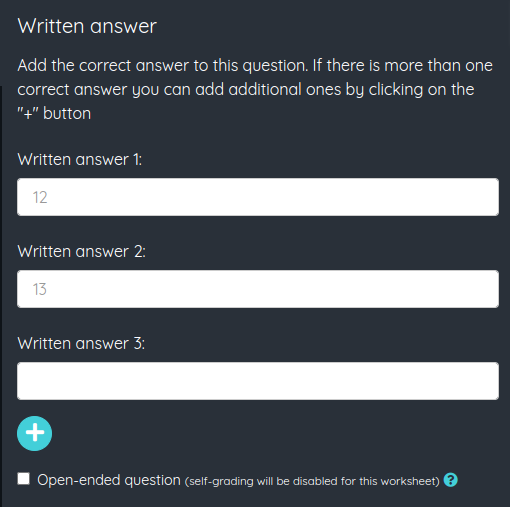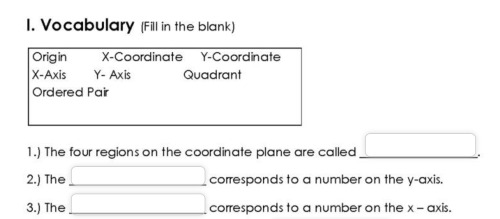The Answer fields must be filled in by the student with the answer, writing what they think is correct. We have two possibilities when adding this type of field: closed questions and open questions. The closed questions will have a series of correct answers that we have to input when creating the field, and can be autocorrected. The open questions do not have a specified correct answer, the teacher will have to correct the question once the student finishes the worksheet.Once an Answer field has been added, its options screen will appear. We will have to input the correct answer or answers. Several answers can be set to adapt to different ways of answering by the students. For example, if the question is "10 / 4" we could input the answers as "2.5", "2,5", "two and a half" and "two point five" and all of them would be counted as valid when autocorrecting the worksheet.

To add more options to the correct answer we must click the + button. We can also add new options with the keyboard, by pressing Ctrl+Intro (or ⌘+Intro in Mac). The editor will display the field filled in with the first of the correct answers.

If we mark the open-ended check this will be an open-ended question and we won't be able to add any answers, also the worksheet won't be self-graded and the question will have to be corrected manually.Here you can see a video showing how a student will see the Written answer field:

« Previous
Next »